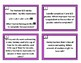# Fraction Review Print and Go Task CardsSubject
Resource Type
File Type

PDF

(324 KB)
Product Rating
Standards
• Product Description
• StandardsNEW

Fraction Review Print and Go Task Cards with answer sheet and key included.

Includes all of the following content:

- Interactive Practice

- Fractions on a number line

- Mixed Numbers/Improper Fractions

- Equivalent Fractions and Simplest Form

- Comparing Fractions

Print on card stock and cut out each task cards. These cards can be used as an interactive game/ scoot, or posted around the room for review.

Solve word problems involving addition and subtraction of fractions referring to the same whole, including cases of unlike denominators, e.g., by using visual fraction models or equations to represent the problem. Use benchmark fractions and number sense of fractions to estimate mentally and assess the reasonableness of answers. For example, recognize an incorrect result 2/5 + 1/2 = 3/7, by observing that 3/7 < 1/2.
Apply and extend previous understandings of multiplication to multiply a fraction by a whole number.
Solve word problems involving addition and subtraction of fractions referring to the same whole and having like denominators, e.g., by using visual fraction models and equations to represent the problem.
Add and subtract mixed numbers with like denominators, e.g., by replacing each mixed number with an equivalent fraction, and/or by using properties of operations and the relationship between addition and subtraction.
Decompose a fraction into a sum of fractions with the same denominator in more than one way, recording each decomposition by an equation. Justify decompositions, e.g., by using a visual fraction model. Examples: 3/8 = 1/8 + 1/8 + 1/8; 3/8 = 1/8 + 2/8; 2 1/8 = 1 + 1 + 1/8 = 8/8 + 8/8 + 1/8.
Total Pages
N/A
Included
Teaching Duration
N/A
Report this Resource to TpT
Reported resources will be reviewed by our team. Report this resource to let us know if this resource violates TpT’s content guidelines.